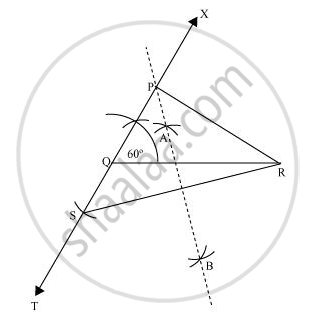# Construct a triangle PQR in which QR = 6 cm, ∠Q = 60° and PR − PQ = 2 cm - Mathematics

Construct a triangle PQR in which QR = 6 cm, ∠Q = 60° and PR − PQ = 2 cm

#### Solution

The below given steps will be followed to construct the required triangle.

Step I:- Draw line segment QR of 6 cm. At point Q, draw an angle of 60°, say ∠XQR.

Step II:- Cut a line segment QS of 2 cm from the line segment QT extended in the opposite side of line segment XQ. (As PR > PQ and PR - PQ = 2 cm). Join SR.

Step III:- Draw perpendicular bisector AB of line segment SR. Let it intersect QX at point P. Join PQ, PR.

ΔPQR is the required triangle.Concept: Some Constructions of Triangles
Is there an error in this question or solution?
Chapter 11: Constructions - Exercise 11.2 [Page 195]

#### APPEARS IN

NCERT Class 9 Maths
Chapter 11 Constructions
Exercise 11.2 | Q 3 | Page 195

Share